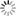# Episode List

OR

### 1995

16 Sep. 1995
Heat 1Be the first one to add a plot.

23 Sep. 1995
Heat 2Be the first one to add a plot.

30 Sep. 1995
Heat 3Be the first one to add a plot.

7 Oct. 1995
Heat 4Be the first one to add a plot.

14 Oct. 1995
Heat 5Be the first one to add a plot.

21 Oct. 1995
Heat 6Be the first one to add a plot.

28 Oct. 1995
Heat 7Be the first one to add a plot.

4 Nov. 1995
Heat 8Be the first one to add a plot.

11 Nov. 1995
Quarter Final 1Be the first one to add a plot.

18 Nov. 1995
Quarter Final 2Be the first one to add a plot.

25 Nov. 1995
Quarter Final 3What will Kylo Ren's crossguard lightsaber look like? What's up with the new dish on the Millennium Falcon? How sweet are the new Stormtrooper helmets? Join Holly Frey from HowStuffWorks as she experiences the beautiful props and costumes from the upcoming Star Wars: The Force Awakens. And make sure to check out all of our coverage from Star Wars Celebration 2015.

2 Dec. 1995
Quarter Final 4Be the first one to add a plot.

9 Dec. 1995
Semi Final 1Be the first one to add a plot.

16 Dec. 1995
Semi Final 2Be the first one to add a plot.

23 Dec. 1995
Grand FinalBe the first one to add a plot.

7 Jan. 1995Be the first one to add a plot.

14 Jan. 1995Be the first one to add a plot.

21 Jan. 1995Be the first one to add a plot.

28 Jan. 1995Be the first one to add a plot.

4 Feb. 1995
International Gladiators 1: Semi Final 1Be the first one to add a plot.

11 Feb. 1995
International Gladiators 1: Semi Final 2Be the first one to add a plot.

18 Feb. 1995